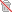게시판 즐겨찾기
편집
드래그 앤 드롭으로
즐겨찾기 아이콘 위치 수정이 가능합니다.
c++ 에서 특수 문자 어떻게 지우나요?
게시물ID : programmer_23279짧은주소 복사하기
작성자 : 초급인
추천 : 1
조회수 : 1151회
댓글수 : 5개
등록시간 : 2021/08/25 15:05:39
 옵션본인삭제금지
#include<iostream>
#include<fstream>
#include<sstream>
#include<string>
#include<iomanip>
usingnamespace std;
constint ArrayMax = 50000;

{
ifstream infile;
string line;
string fileName;
cout << "Type the name of text to read ";
cin >> fileName;
cout << "Opening File: " << "\""<< fileName <<"\""<<endl;
infile.open (fileName); //name of file here. plz mention Complete path if file is not at root
int count=0;
string data;
if (infile.is_open()) //if file opened
{
while(infile>>data)
{
words[count] = data;
count++;
}
return count;
}
{
cout << "Sorry, we could not find the file." << endl;
}
infile.close();
return -1;
}

void removeSpecialCharacter(string s)
{
for (int i = 0; i < s.size(); i++)
{
// Finding the character whose
// ASCII value fall under this
// range
if (s[i] < 'A' || s[i] > 'Z' && s[i] < 'a' || s[i] > 'z')
{
// erase function to erase
// the character
s.erase(i, 1);
i--;
}
}
}

string cleanWord(string in)
{
string data = "";
char ch;
for(unsignedint i = 0; i < in.length(); i++)
{
ch = in[i];
//convert upper case to lower
if(ch >= 'A' && ch <= 'Z' )
{
data += ch-'A'+'a';
}
elseif(ch>='0' && ch<='9' )
{
data += ch;
}
elseif(ch>='a' && ch<='z' )
{
data += ch;
}
///////////// else
//////////////// {
/////////////////// data.erase(i, 1);
//////////////// }

}
return data;
}

void cleanAndStoreToFile(string *word,int count)
{
ofstream myfile ("ast1log.txt");
cout << "Exporting data to file......" << endl;
for(int i=0; i < count; i++)
{
myfile << word[i] << " ===> " << cleanWord(word[i]) << endl;
}
}

int main()
{
string words[ArrayMax];

cout << "Array size is : " << ArrayMax << endl;

cleanAndStoreToFile(words,count);
cout << "Finished Reading " << count <<" words. Closing File." << endl;

cout << "Commands" << endl;
cout << "Unigram: search for unigram" << endl;
cout << "Bigram : search for bigram" << endl;
cout << "Save : save 1gms.txt and 2gms.txt" << endl;
cout << "Index : print index of unigram or bigram " << endl;
cout << "Quit/q : terminate program" << endl;
}

string cleanWord(string in)
에서
파일 읽으면
일단 숫자 포함 대문자 소문자로 빠꾸고 싶은데 어렵네요....

파일 읽는게
*** START OF THIS PROJECT GUTENBERG EBOOK THE TIME MACHINE *** Release Date: October 2, 2004 [EBook #35] Author: H. G. Wells

이라면
*** ===> START ===> start OF ===> of THIS ===> this PROJECT ===> project GUTENBERG ===> gutenberg EBOOK ===> ebook THE ===> the TIME ===> time MACHINE ===> machine *** ===> Release ===> release Date: ===> date October ===> october 2, ===> 2 2004 ===> 2004 [EBook ===> ebook #35] ===> 35 Author: ===> author H. ===> h G. ===> g Wells ===> wells

자꾸 이렇게 되네요.

START ===> start OF ===> of THIS ===> this PROJECT ===> project GUTENBERG ===> gutenberg EBOOK ===> ebook THE ===> the TIME ===> time MACHINE ===> machine Release ===> release Date: ===> date October ===> october 2, ===> 2 2004 ===> 2004 [EBook ===> ebook #35] ===> 35 Author: ===> author H. ===> h G. ===> g Wells ===> wells
원래라면 이렇게 되야하는데....

도움 부탁드려요전체 추천리스트 보기
새로운 댓글이 없습니다.
새로운 댓글 확인하기공지 운영 게시판요청 자료창고